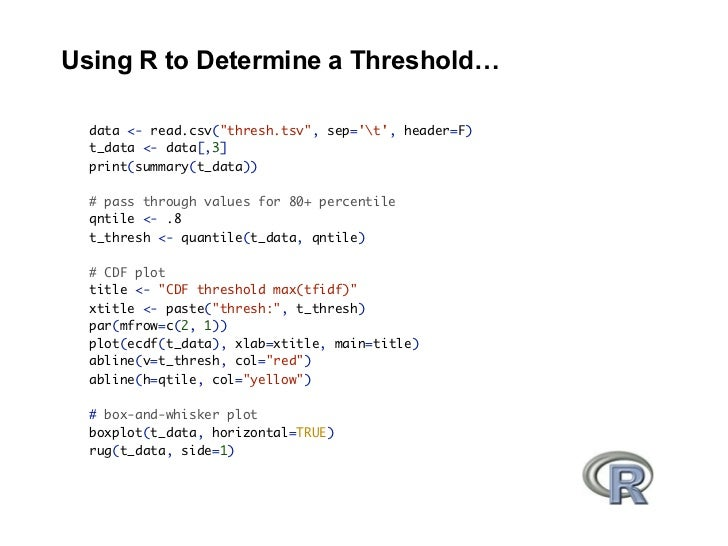Successfully reported this slideshow.Upcoming SlideShare
×

# Using R to Determine a Getting Started on Hadoop

20,793 views

Published on

Using R to Determine a Threshold…

t_data <- data[,3]
print(summary(t_data))

# pass through values for 80+ percentile
qntile <- .8
t_thresh <- quantile(t_data, qntile)

# CDF plot
title <- "CDF threshold max(tfidf)"
xtitle <- paste("thresh:", t_thresh)
par(mfrow=c(2, 1))
plot(ecdf(t_data), xlab=xtitle, main=title)
abline(v=t_thresh, col="red")
abline(h=qtile, col="yellow")

# box-and-whisker plot
boxplot(t_data, horizontal=TRUE)
rug(t_data, side=1)

Published in: Technology
• Full Name
Comment goes here.

Are you sure you want to Yes No